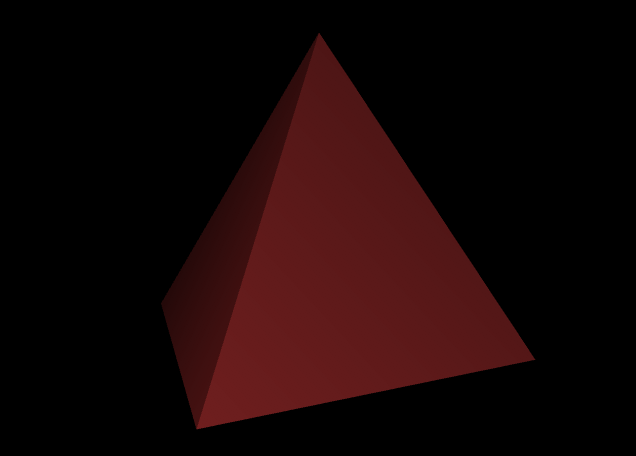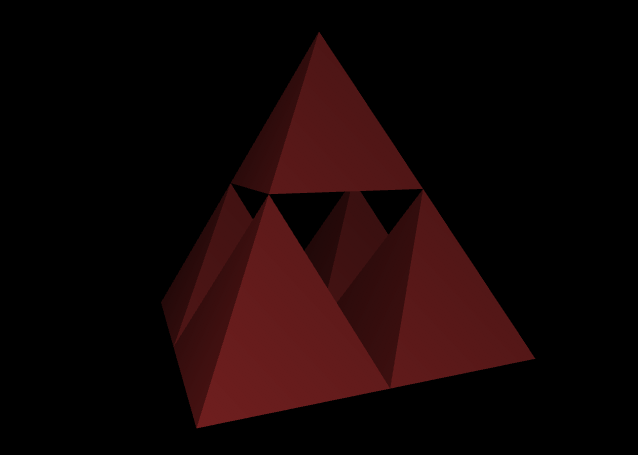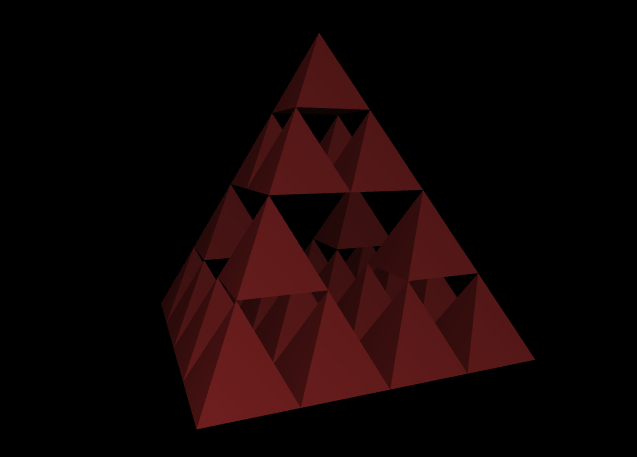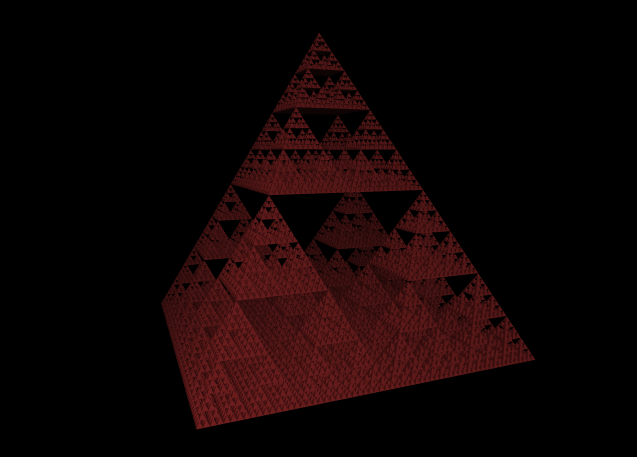«

# rendering a sierpinski pyramid in clojure

### 2009

A three-dimensional Sierpinski triangle is created recursively, by replacing each pyramid with five sub-pyramids. To create one, we use Penumbra, which is a thin but idiomatic wrapper for OpenGL.

First, we create a pyramid.

``````(defn draw-pyramid []
(material 0.8 0.2 0.2 1)

;; draw the four sides
(draw-triangle-fan
(vertex 0 1 0)
(dotimes [_ 5]
(rotate 90 0 1 0)
(normal 1 0.5 1)
(vertex 0.5 0 0.5)))

;; draw the bottom
(normal 0 -1 0)
(dotimes [_ 4]
(rotate -90 0 1 0)
(vertex 0.5 0 0.5))))
``````

The pyramid is defined in two parts: a fan of triangles around the tip, and a square at the bottom. Both are defined by rotating iteratively around the central axis.

``````(draw-pyramid)
``````Next, we turn one pyramid into five smaller pyramids.

``````(defn subdivide [display-list]
(push-matrix
(scale 0.5 0.5 0.5)

;; draw the top sub-division
(push-matrix
(translate 0 1 0)
(call-display-list display-list))

;; draw the bottom four sub-division
(dotimes [_ 4]
(rotate 90 0 1 0)
(push-matrix
(translate 0.5 0 0.5)
(call-display-list display-list)))))
``````

The subdivide function takes a display list as its argument, which is a cached list of OpenGL calls. This list is called five times, first at the top and then four times around the central axis.

``````(subdivide (get-display-list (draw-pyramid)))
``````We can call subdivide as many times as we like, but Clojure gives us a superior approach, by way of lazy sequences.

``````(defn sierpinski []
(iterate
#(get-display-list (subdivide %))
(get-display-list (draw-pyramid))))
``````

The `iterate` function creates a lazy sequence based on a function that takes n and returns n+1. On each iteration, the previous display list is shrunk to half its size on all dimensions, and drawn five times. This lets us generate as much geometry as we need, and no more.

``````(call-display-list
(nth (sierpinski) 2))
````````````(call-display-list
(nth (sierpinski) 6))
``````Complete code can be found in the `/examples` subfolder in the Penumbra repository.